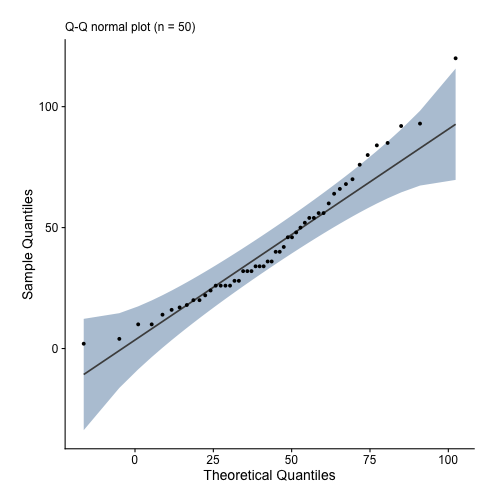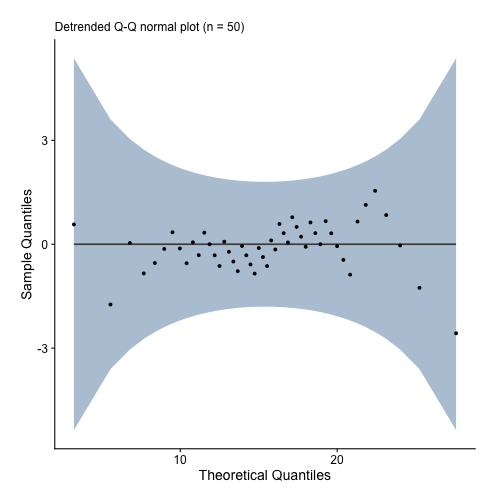by Guangming Lang

• r

### Updated October 4, 2018

In an earlier post, I showed you how easy it is to make high quality histograms and boxplots using ezplot. When we’re analyzing a continuous variable, we often want to check if it’s normally distributed. Q-Q plot is a good tool to do that.

Make sure you first install ezplot by running the command devtools::install_github("gmlang/ezplot").

We’ll use the cars dataset, which comes with the base R distribution. It has two variables, speed and dist. Both are continuous. Let’s first create a normal Q-Q plot for dist.We see dist is approximately normal because most of the data points are aligned linearly along the 45 degree diagonal line and within the confidence band. Next, we make a detrended normal Q-Q plot for speed and observe it’s also normally distributed because all data points are randomly scattered around $y = 0$ and within the confidence band.If you liked these how-to blog posts, you may want to check out my ezplot book.